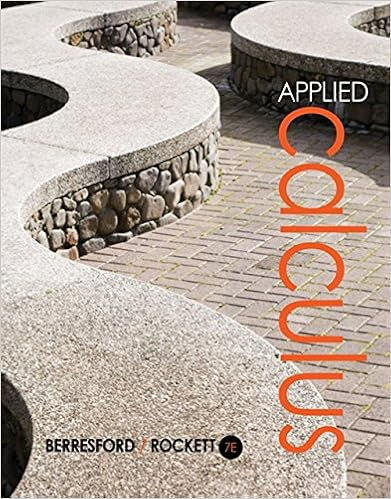# Marks recall that the maclaurin series of the sine

• Test Prep
• 7
• 75% (4) 3 out of 4 people found this document helpful

This preview shows page 7 out of 7 pages.

##### We have textbook solutions for you!
The document you are viewing contains questions related to this textbook.The document you are viewing contains questions related to this textbook.
Chapter 3 / Exercise 56
Applied Calculus
Berresford/RockettExpert Verified
4. (5 marks) Recall that the Maclaurin series of the sine function is cos x22420( 1)1...( 1)...(2 )!2!4!(2 )!nnnnnxxxxnn=-=-+-+ -+. (a) (2 mark) Find the Maclaurin series of the function y= cos (x2(b) (3 marks) Find the Maclaurin series of a function y= F( x) defined by definite integral F(x) = cos()xtdt. = ). 20Solution
##### We have textbook solutions for you!
The document you are viewing contains questions related to this textbook.The document you are viewing contains questions related to this textbook.
Chapter 3 / Exercise 56
Applied Calculus
Berresford/RockettExpert Verified
•••